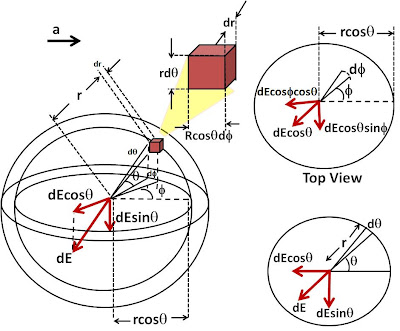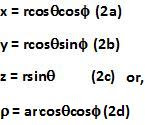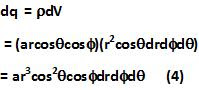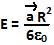## Sunday, March 21, 2010

### Irodov Problem 3.18Without loss of generality, let us consider a coordinate system where, the the x-axis is aligned in the direction of the vector.

In that case,As seen from the figure, in the spherical coordinate system,Consider an infinitesimally small section of the sphere (the cuboid shown in the picture) located at a distance r from the center, at an polar angleand azimuthal anglewhile spanning a width dr,a polar angleand an azimuthal angle ofas shown in the picture. A shown in the picture the volume of the infinitesimally small section has a volumeThe total charge contained in this infinitesimally small section is then given by,The electric field due to this infinitesimally small section of the sphere at the center of the sphere will be given by,But at the beginning of the problem to solve the problem we assumed a coordinate system whose x-axis was aligned in the direction of the vector. Hence, in the original coordinate system we can write,